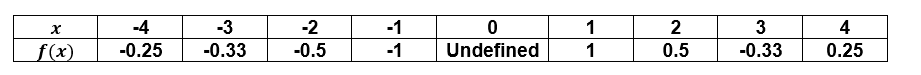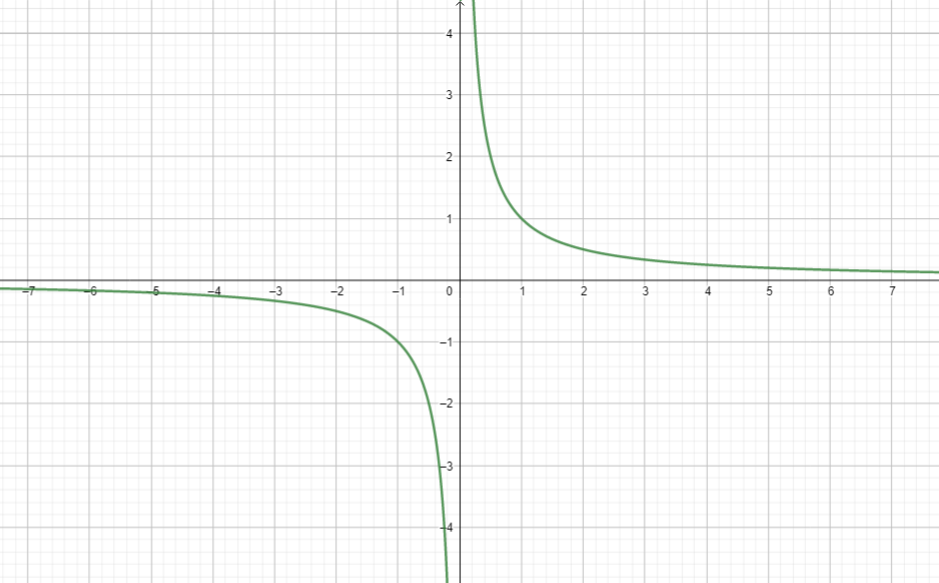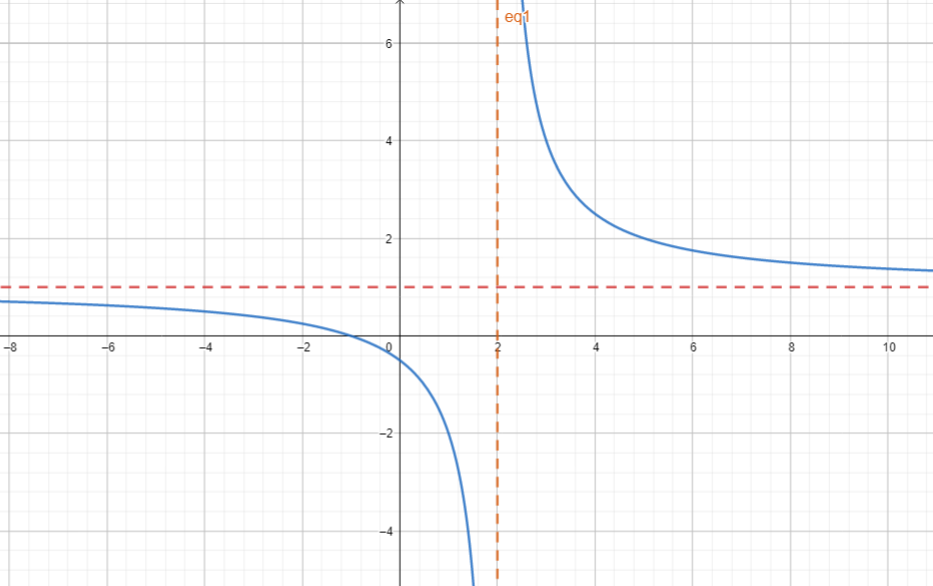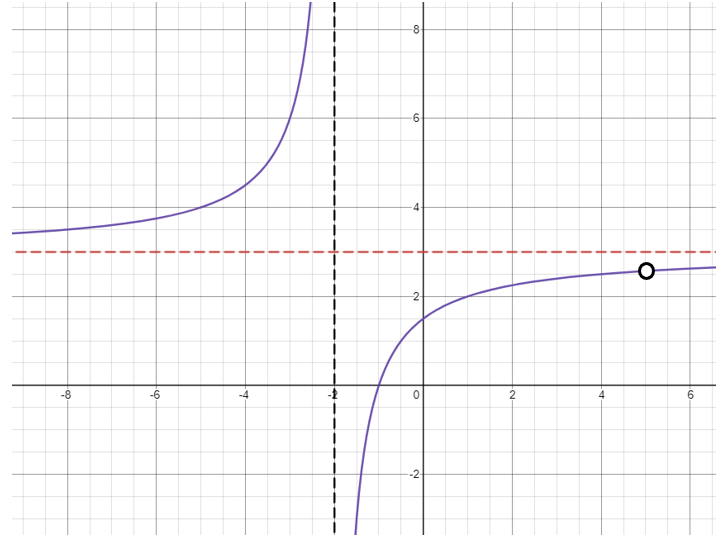# Algebra 2 - Rational Functions

## Introduction

• Rational Function is a function in the form of  are both polynomial functions. It must be noted that  have no common factors except 1.
• In general, a rational function is written in the form $f\left(x\right)=\frac{{a}_{n}{x}^{n}+{a}_{n-1}{x}^{n-1}+...+{a}_{1}x+{a}_{0}}{{b}_{m}{x}^{m}+{b}_{m-1}{x}^{m-1}+...+{b}_{1}x+{b}_{0}}$ since both the numerator and denominator are polynomial functions.
• Recall the definition of a polynomial: a sum of a finite number of monomials whose terms are the product of any real number and variable with nonnegative integral exponents.
• A polynomial function is expressed in the form
• The domain is the set of all $x$ real numbers for which the denominator is not equal to 0.
• Continuity of Rational Functions - All rational functions are continuous for all real numbers except at those values where the denominator is 0.
• Every polynomial function is a rational function with a denominator $q\left(x\right)=1$. It is also similar to the concept or definition of rational expressions and rational numbers for having a denominator of 1.

Example 1. The function $f\left(x\right)=5x-1$ is rational because it is a polynomial (specifically linear) whose denominator is 1

Example 2. The function $g\left(x\right)=\frac{{x}^{-2}+1}{{x}^{-2}-1}$ is rational. Although it may seem that it's not rational, this can be simplified to $g\left(x\right)=\frac{{x}^{2}+1}{1-{x}^{2}}$.

Example 3. The function $f\left(x\right)=\frac{1}{\sqrt{x}+1}$ is not rational because $\sqrt{x}+1$ is not a polynomial function.

Example 4. The function $f\left(x\right)=\frac{4x+28}{x+7}$ is rational because this is simplified to $f\left(x\right)=4$

Example 5. The equation $y\left(4x+1\right)=\frac{\left(2x-3\right)y}{x}$ does not represent a rational function because this is further simplified to $x=\frac{2x-3}{4x+1}$ which consist of only a single variable. There is no dependent variable to establish the concept of function.

## Properties and Representations of Rational Function

• Rational functions can be represented using equations, tables of values, and graphs.

Example 1. Represent  using a table of values.

Solution.Graph:Domain and Range of Rational Functions

• The domain of rational functions is the set of all real numbers except for values that make the denominator equal to 0.
• To solve for the range, we express the function equation in terms of $y$ and determine the restricted values in the denominator.

Example 1. What is the domain and range of $f\left(x\right)=\frac{x+1}{5-x}$?

Solution:

To find the domain, we equate the denominator $5-x$ to 0 and solve for $x$

$5-x=0\to x=5$

Thus, the domain is the set of all real numbers except $x=5$ or this can be written as .

To find the range, we have:

$y=\frac{x+1}{5-x}\to 5y-xy=x+1\to -xy-x=-5y+1\to -x\left(y+1\right)=1-5y$

The equation for x in terms of y is $x=\frac{5y-1}{y+1}$. Solving for the restricted value in the denominator gives a range of

Example 2. For cases like $y=\frac{x+3}{{x}^{2}-5x+6}$, the domain is the set of all reals except

To solve for the range, this can be done using a graphical method or algebraic procedure.

In using the algebraic procedure, we rewrite the entire equation as $x{y}^{2}-x\left(5y+1\right)+6y-3=0$

Using the quadratic formula, this gives $x=\frac{5y+1±\sqrt{{y}^{2}+22y+1}}{2y}$

Focusing on the radicand ${y}^{2}+22y+1\ge 0$, the range is .

## Intercepts, Asymptotes, and Graphs

• Graphically speaking, an asymptote is a straight line (or curve line) that the graph of a function gets closer to but does not touch.
• An asymptote is a line approached by the graph of a function as $x$ increases or decreases without bounds.
• A vertical asymptote is the line $x=a$ wherein the graph increases or decreases without bound on one or both sides of the line as $x$ moves closer and closer to $a$.
• The horizontal asymptote is the line $y=b$ that is being approached by the graph as $x$ increases or decreases without bounds. Notice that it doesn't have to approach $y=b$ as $x$ both increases and decreases. It only needs to approach one side for it to be a horizontal asymptote.
• An oblique asymptote is obtained when the numerator of $f\left(x\right)$ has a degree that is one degree higher than the degree of the denominator.
• Intercepts are x-coordinates or y-coordinates of the points at which a graph cross the x-axis or y-axis, respectively. The x-intercepts represent the zeros of the function.

Example 1. Study the graph of .Solution:

The behavior of the graph above is described as follows:

1. $f\left(x\right)\to -\infty$ as $x\to {0}^{-}$

This implies $f\left(x\right)$ decreases without limit as $x$ approaches 0 from the left.

2. $f\left(x\right)\to \infty$ as $x\to {0}^{+}$

This means $f\left(x\right)$ increases without bound as $x$ approaches 0 from the right.

Example 2. What are the x-intercept, y-intercept, and asymptotes of the function $g\left(x\right)=\frac{x+1}{x-2}$? Sketch its graph.

Solution:

To solve for the vertical asymptote, we solve for x when the denominator is equated to 0.

In this case, we have $x-2=0\to x=2$

When the degree of the numerator and denominator are equal, the horizontal asymptote is determined by getting the ratio of the numerical coefficients of the leading terms in the numerator and denominator.

In this case, we have: $g\left(x\right)=y=\frac{1}{1}\to y=1$

To find the x-intercept, we have:

To solve for the y-intercept, we have:

Graph:## Cheat Sheet

• To completely decribe asymptotes, let $f\left(x\right)=\frac{p\left(x\right)}{q\left(x\right)}=\frac{{a}_{n}{x}^{n}+{a}_{n-1}{x}^{n-1}+...+{a}_{1}x+{a}_{0}}{{b}_{m}{x}^{m}+{b}_{m-1}{x}^{m-1}+...+{b}_{1}x+{b}_{0}}$ where  have no common factors except, and $q\left(x\right)\ne 0$.
• The vertical asymptotes of $f$ are the lines $x=a$ where $a$ is a real zero of $q\left(x\right)$ after simplification of the $f\left(x\right)$ in lowest terms.
• If $n, then the function has a horizontal asymptote $y=0$ (x-axis).
• If $n=m$, then the function has a horizontal asymptote $y=\frac{{a}_{n}}{{b}_{m}}$. This is when  having the same degree.
• If $n>m$, then the function has no horizontal asymptote.
• If $n-m=1$ and $f\left(x\right)$ can be simplified to a form $f\left(x\right)=kx+v+\frac{r\left(x\right)}{q\left(x\right)}$, then the line $y=kx+v$ is the oblique asymptote. The expression  are real numbers, is the quotient when we divide the numerator by the denominator.
• If and $f\left(x\right)$ can be simplified to a form $f\left(x\right)=k{x}^{2}+v+\frac{r\left(x\right)}{q\left(x\right)}$, then the curved line $y=k{x}^{2}+v$ is the parabolic asymptote. The expression $k{x}^{2}+v$ is the quotient when we divide the numerator by the denominator.
• There are two common methods of sketching the graph of rational functions without using Calculus: 1. Using a Table of Values, 2. Using Table of Signs.

## Blunder Areas

• There are cases wherein rational functions intersect the horizontal asymptotes. Study the cases like $y=\frac{3{x}^{2}+2}{{x}^{2}+4x-5}$ or $y=\frac{{\left(x+1\right)}^{2}\left(x-3\right)}{{\left(x+3\right)}^{2}\left(x-2\right)}$.
• It is a common misconception that the graph of a rational function does not intersect the horizontal asymptote or slant asymptote.
• There are cases wherein rational functions have no vertical asymptotes but have horizontal asymptotes. These cases apply when there are no restricted real values in the denominator. Study the cases like .
• Some rational functions have a hole in the graph. This case arises when a rational function is reduced ta o simplified form. The common factor makes the graph contain a hole. Study the graph of $f\left(x\right)=\frac{3{x}^{2}-12x-15}{{x}^{2}-3x-10}$ which is expressed as $f\left(x\right)=\frac{3\left(x+1\right)\left(x-5\right)}{\left(x-5\right)\left(x+2\right)}$. Its simplest form is $f\left(x\right)=\frac{3\left(x+1\right)}{x+2}$. Hence, the hole in the graph is $x=5$. This is not a vertical asymptote. The graph is shown below.